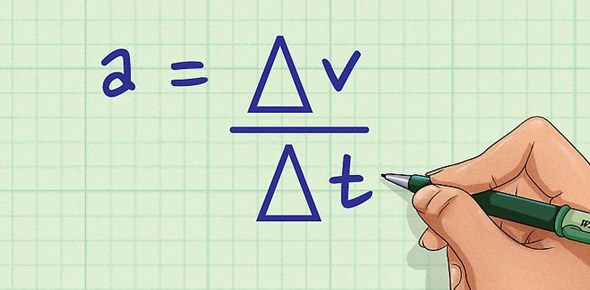# Kinematics: Acceleration

7 Questions | Total Attempts: 73SettingsUse this quiz to determine if you need any additional work on any of the concepts. Please keep in mind that if you do not type the answer in EXACTLY as I have it set up the computer will assume that it is wrong. This means you MUST put in the units! After all, without units the answer technically is wrong! However if it marks it wrong and it is obviously correct keep that in mind when it gives you your score. Remember, this is NOT a graded quiz. It is part of your careers grade to do the quiz, but the score is just a tool for you to determine if you need to do further work. YOUR LEARNING IS UP TO YOU!

Related Topics
• 1.
A car accelerates from 5.0 m/s to 21 m/s at a rate of 3.0 m/s2.  How far does it travel while accelerating?
• A.

69 m

• B.

207 m

• C.

41 m

• D.

117 m

• 2.
An airplane needs to reach a velocity of 203.0 km/h to take off.  On a 2000 m runway, what is the minimum acceleration necessary for the plane to take flight?
• A.

0.79 m/s2

• B.

0.87 m/s2

• C.

0.95 m/s2

• D.

1.0 m/s2

• 3.
Assuming equal rates of acceleration in both cases, how much further would you travel if braking from 56 mi/hr to rest than from 28 mi/hr?
• A.

3.2 times farther

• B.

4 times farther

• C.

4.8 times farther

• D.

5.2 times farther

• 4.
A sports car has an average acceleration of 13.1 miles/hr/sec.  how long does it take for the car to reach 60. mi/hr, if it starts from rest?
• A.

5.5 s

• B.

3.1 s

• C.

4.6 s

• D.

8.8 s

• 5.
A car is traveling north at 17.7 m/s.  After 12 s its velocity is 14.1 m/s in the same direction.  Find the magnitude and direction of the car's average acceleration.
• A.

2.7 m/s2, South

• B.

0.30 m/s2, North

• C.

2.7 m/s2, North

• D.

0.30 m/s2, South

• 6.
Acceleration is sometimes expressed in multiples of g, where g = 9.8m/s2 is the acceleration due to the earth's gravity.  In a car crash, the car's velocity may go from 26 m/s to 0 m/s in 0.15s.  How many g's are experienced, on average, by the driver.
• A.

13 g

• B.

18 g

• C.

22 g

• D.

23 g

• 7.
A train starts from rest and accelerates uniformly, until it has traveled 5.6 km and acquired a velocity of 42 m/s.  The train then moves at a constant velocity of 42 m/s for 420 s.  The train then slows down uniformly at 0.065 m/s2, until it is brought to a halt.  The distance traveled by the train while slowing down, in km, is closest to:
• A.

0.14 m/s2

• B.

0.17 m/s2

• C.

0.19 m/s2

• D.

0.20 m/s2

• E.

0.16 m/s2